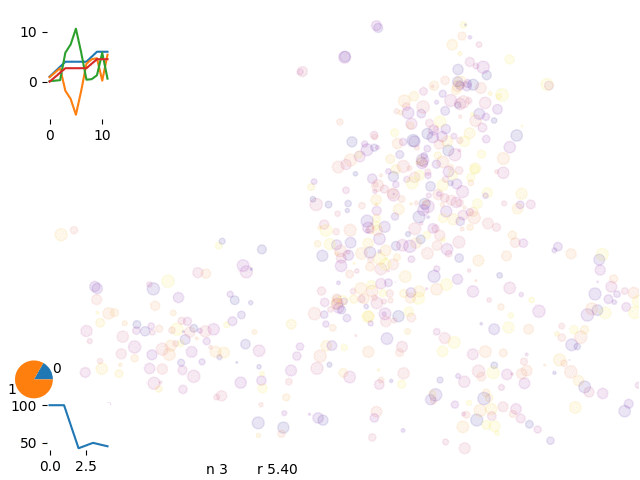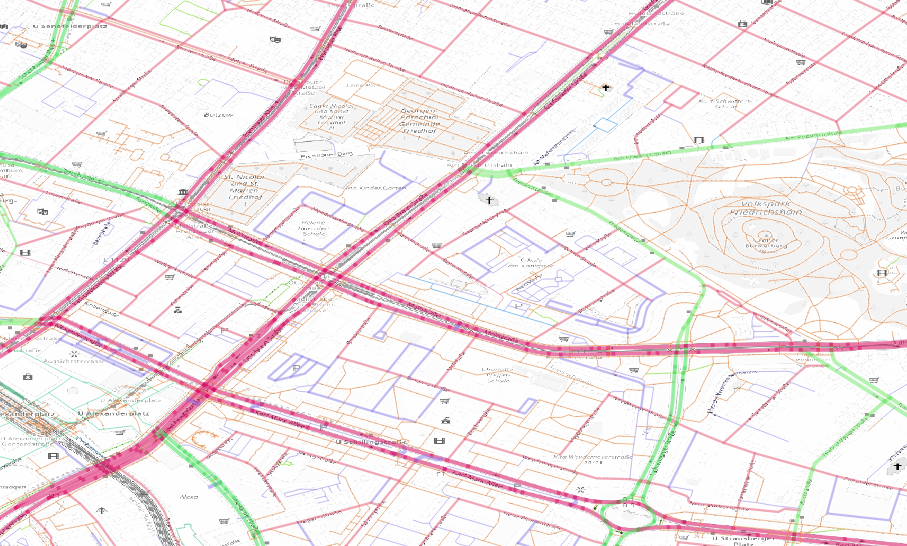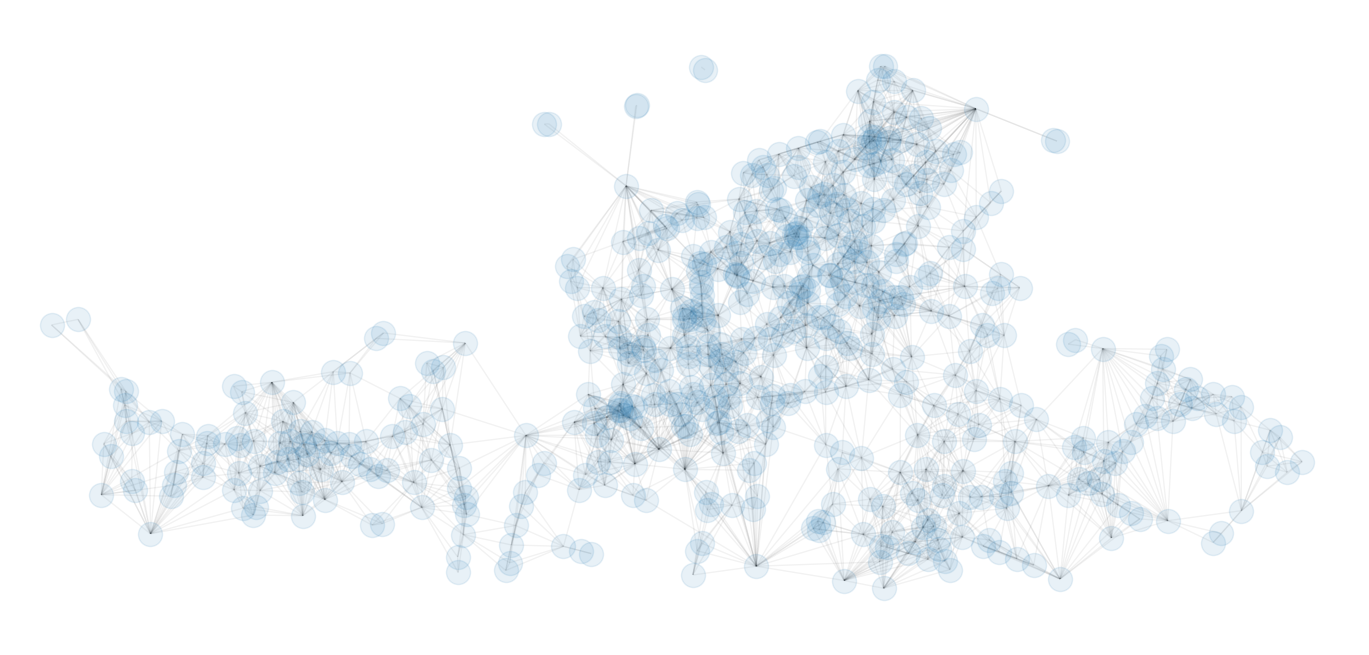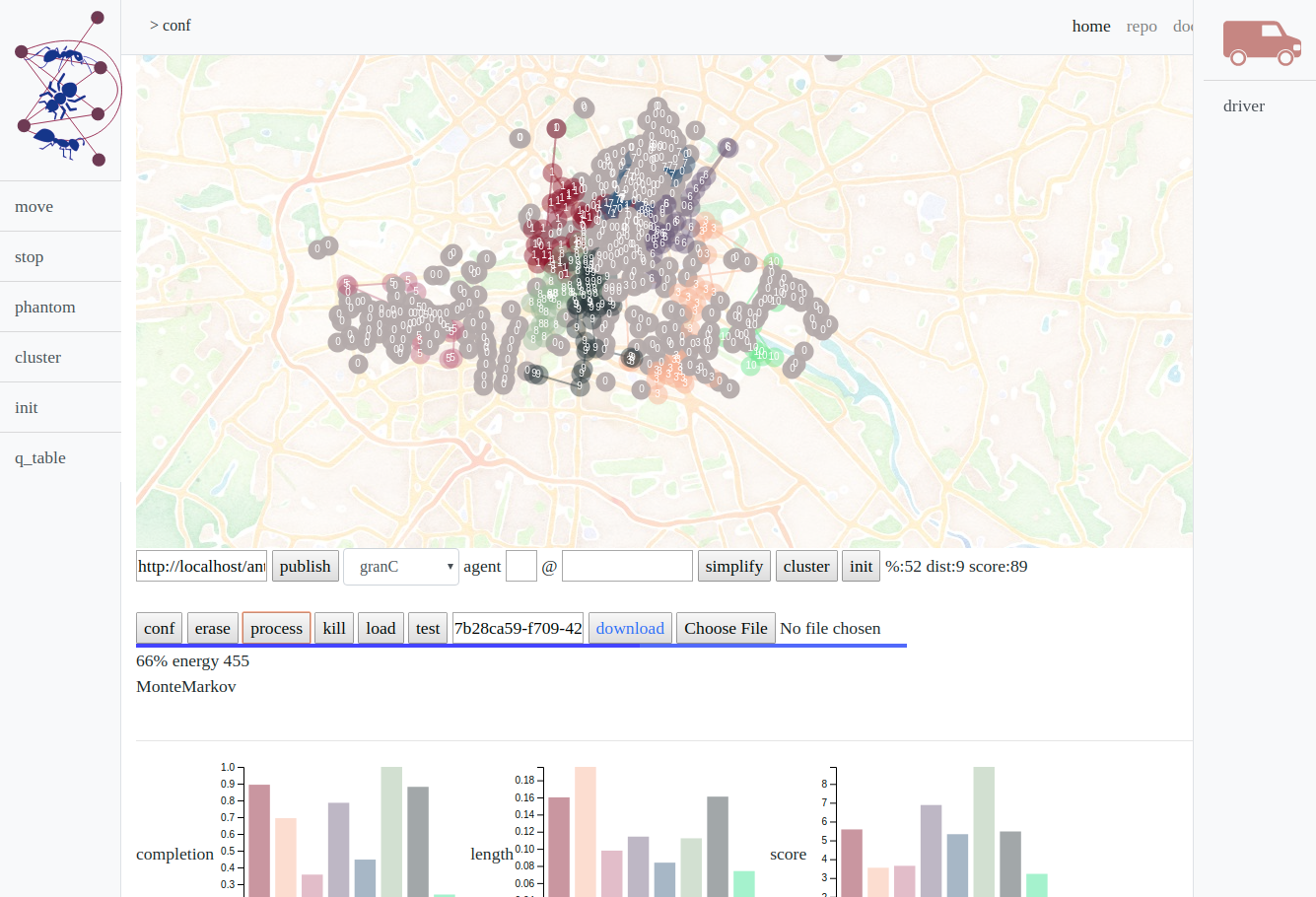# Antani

Ant - agent/network intelligence

ants optimizing paths on a network

## Efficiency

Why operation efficiency?

• Fill the gap between revenue and cost
• Think as a network, not single operator
• Select the most valuable tasks

## Problem

The closest path facing the warehouse fullfilling the most valuables tasks

optimization engine requirements

• Focus on profitability
• Plan shifts
• Consider task time and revenue

## Commercial softwares?

Delivery based, routific: * Long deviations * Skipped tasks * Unclear priorities

problems with routific

No resuming, everytime a new simulation

open source software suite for optimizationor-tools solutions

Many crossing Incomplete vans, long trajectories:

• incomplete solution (-20%)
• job to be killed
• 0.5-75mins

## In house solutionin house optimization engine

## Path optimization

Path like polymers

PhD defense – computational biophysics 2012

## Optimization engine

Ludewa/Tanzania - 2013

Electrical line design to connect households to the new power plant

## Building a graph

From detailed street network to an efficient graphSubset, connect, simplify, subgraph, check directions…

## weighting

Weight every segment

Maxspeed, streetclass, length, junctions

## Graph setup

Checking routes

checking routes

Good correlation between spot2spot routes in graphs and air distance

Osrm – open street routing machine

## Routes

Subset the city in geohashes (~70m)

routing information

Calculate all pair distances and build a lookup database

pair relationship database

Sum up tasks in the same geohash

graph edges kept

Keep only neighbor connections between tasks

# Sense move reward

Ant/colonyan ant per loop, iterate over the networkenergy definition

Energy: * +separation * +task value * - area * - task time * - tot distance

antani concept

## Path opt

optimize sequences

## Monte Carlo

Single random move

energy evolution

Asyntotic energy and move acceptance rate evolution

## Markov Chainsmarkov chains

We limit the possible moves leaving the most probable

## Enhance moves

single, Markov, distance, extrude

move selection

Spot selection according different probabilities

## Simulation boost

• Faster convergency
• Higher acceptance

Single move, routific optimization, Markov chain, extrusion, grand canonical…

## Calculation time

Early simulations were too slow

## Find a score:

scoring

kpi comparison

+completion + revenue – distance - time

## Reinforcement learning

Improve acceptance

reinforce moves

Single agent reinforcement is too slow and chaotic

## Posterior probability

Improve with real data

posterior probabilities

demand forecast

## Microservice design

Backbone +microservices

engine design

## Asynchronicityantani infrastructure

Client – broker/worker design

## Frontend

OpenLayers, d3, ajaxDocumentationantani frontend

## Code

library ecosystem

…started in 2006

## Outlook

• Drivers feedback
• Process real data
• Compare with rideos

## Summary

• Start, stop, resume
• First draft within few seconds
• Clear operating areas
• Weighting potential revenues
• Focus on profitability

## Acknowledgement

Circ – fleet engine team Carlo Mazzaferro – productization of antani

# Theory

## Gibbs sampling

We describe a probability distribution via its moments μ⃗

p(x⃗; μ⃗)

We have a system x⃗ where each x is in a certain state s. We define a energy function which depends on the states of system and a set of parameters θ. In our case the system is a series of field tasks on a map and the state is the agent who is fulfilling the task.

The energy of the system is the sum of the revenue per task minus the cost: task time and path length. The parameter set θ defines the revenue and cost factor + external factors (temperature T, traffic time h,…). Ideally we will express the parameter set in terms of external factors θ(T, h) or change the metric (distance) of the system d(T, h)

Ea(x⃗|θ) = ns ⋅ rs − cd ⋅ da − ns ⋅ ts

where ns is the number of spots, rs the total revenue per spot, ts is the total operation time, da the distance of that agent.

The probability distribution for a certain state and parameter follows the Boltzmann distribution

$$p(|) exp(-E()/kT) Target probability distribution$$ p(\vec{x}) = \frac{w(\vec{x})}{Z} = \frac{1}{Z} \prod_c \phi_c(x)$$estimator$$ \frac{1}{T} \sum_{t=1}^{T} \phi(\vec{x}) \qquad E_{p(x)}|\phi(x)| = \sum_x p(x)\phi(x) $$From the state x⃗ we create a state x⃗ where we create a sample xj → xj, basically: x⃗′ = x1, x2, ..., xj′, ..., xn$$ p(x) = \frac{exp(E(x)/T)}{Z}  A(x'|x) = min(1,p(x')/p(x)) = min(1,exp(\frac{ E(x') - E(x)}{T})) $$## Bayesian statistics We want to calculate the posterior probability doc which is the probability of a parameter set θ from a given state X$$ p(\theta|x) = \frac{l(x|\theta)p(\theta)}{p(x)} $$where l(x|θ) likelihood, p(θ) prior, p(x′|x) the probability to move from state x to state x and p(X) normalization factor p(X) = ∫dθ * p(X|θ * )p(θ * ) The likelihood is about finding the momenta of the distribution for a given data set (usually via regression), the probability distribution is the theoretical distribution for the system (independent on the data acquired). In a correct sampling the two match. proposal distribution p(x) - target distribution g(xp(θ|X) Step increment θ′ = θ + Δθ$$\rho = \frac{g(\theta'|X)}{g(\theta|X)} \qquad \rho = \frac{p(X|\theta')p(\theta')}{p(X|\theta)p(\theta)}$$sampling from probability from a state x doc xπ̃(x) High dimensional computing (over all states) c = E[f(x)] = ∫π(x)f(x)ds optimization x * = argmaxπ(x) Learning and Bayesian hierarchical modeling for a given parameter set Θ$$ \Theta * = argmax l(\Theta) ; l(\Theta) = \sum_{i=1}^{n} log p(x_i;\Theta)